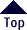COROLLARIES
to
THE  BRUNARDOT  THEOREM

 A = Alpha (motion = y-axis) l = Light
 a = apogee M = Mean (s2 / v)
 c = chord, major o = opposing wave crest
 d = diagonal p = perigee
 E = Energy, Internal r = radius
 f = Force s = soliton
 H = Harmonic T = Tau (time, etc. = x-axis)
 HR = Harmonic Ratio CU = Conceptual Unit
 i = Brunardot Iteration v = vector
 I = Infinity line (x-axis) w = wave crest
 inc. = increment between "i"s x = foci
 K = Unimetric Factor
 d = Natural Prime number (Nature's Scale)
 r = Odd Integer
 L = Integer divisible by four
 a, d, L, p, r, s, v and c2 are integers.
 p, s, v and a are consecutive terms of a series.
 p is a function of Alpha and Tau.
 s is a function of Tau.
 An Infinity line, I, is infinite in length
 and infinitesimal in width.
 Corollary formulas to Brunardot's Theorem:
 p = f(A,T)
 s = f(T)
 r = 2p - 1
 L = 2s
 d = L + 1
 v = p2
 a = 2p2 - p
 c2  = 2v2 - s2
 e = v2 - s2
 x = p/s
 F = square root of e
 And, thus:
 U = d - Lv = s + p
 U = 2p - r
 v = s + p
 r = 2v - d
 c2  = f2 + v2
 d + r = L + 2p
 a = 2s + p
 e = ( c2 - s2)/2
 E-mail :  Brunardot@Brunardot.com
 There is one Universe.
 It is perpetual, in equilibrium;
 and, a manifestation of the Unified Concept; thus; . . . the Fundamental Postulate.
 also,
 are a single discipline, Philogic, which proclaims perpetuity
 and the nexus of Life; such is
 . . . Conceptualism.Terms of:  ©Copyright 1999-2015 by Brunardot

000101 0:01 am